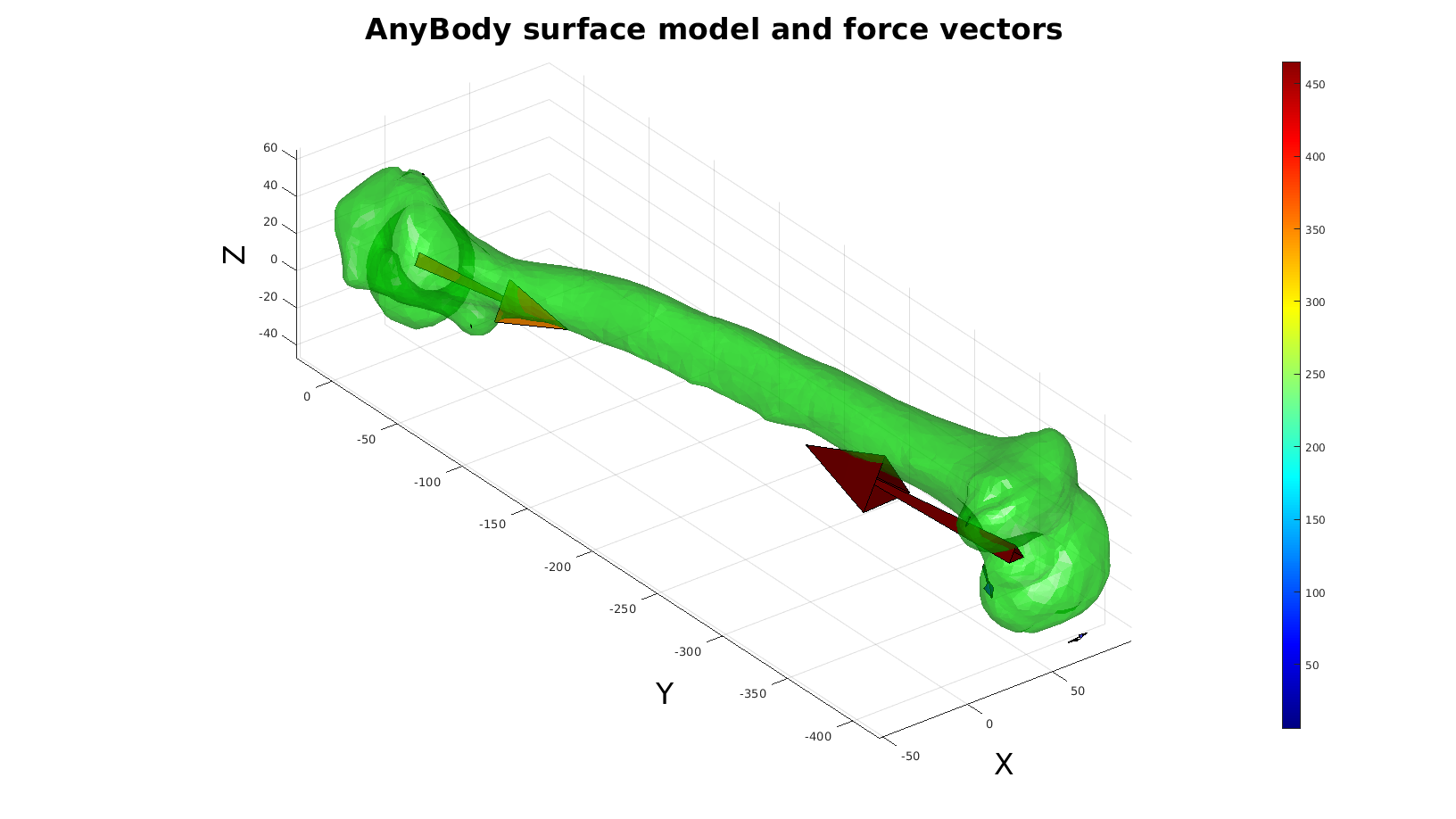# importAnyBodyOutput

Below is a demonstration of the features of the importAnyBodyOutput function

## Contents

```clear; close all; clc;
% warnings off;
```

Plot settings

```faceAlpha=1;
fontSize=25;
markerSize=25;
cMap=jet(250);
```
```%Set main folder
defaultFolder = fileparts(fileparts(mfilename('fullpath')));
```

## Import AnyBody analysis data

```pathName_TXT=fullfile(defaultFolder,'data','AnyBody');
fileName=fullfile(pathName_TXT,'femurData.txt');
structOut = importAnyBodyOutput(fileName);
```

## Import AnyBody STL file as patch data to aid visualization

```pathName_STL=fullfile(defaultFolder,'data','STL');
fileName=fullfile(pathName_STL,'femur.stl');
[stlStruct] = import_STL_txt(fileName);
```

Plotting the models

```pColors=autumn(numel(stlStruct.solidNames));

cFigure;
title('AnyBody surface model and force vectors','fontSize',fontSize);
xlabel('X','fontSize',fontSize);ylabel('Y','fontSize',fontSize); zlabel('Z','fontSize',fontSize); hold on;
for q=1:1:numel(stlStruct.solidNames)
F=stlStruct.solidFaces{q};
V=stlStruct.solidVertices{q};
V=V*1000;
patch('Faces',F,'Vertices',V,'FaceColor','g','EdgeColor','none','FaceAlpha',0.5);
end
view(3); axis equal; axis tight; axis vis3d; grid on;
lighting phong;
drawnow;

% Access and visualize force data

F_vec=structOut(1).F; %Force vectors
Fm=sqrt(sum(F_vec.^2,2));
V_vec=structOut(1).Pos; %Position vectors
V_vec=V_vec*1000; %Conver to meters

logicPlot=Fm>(max(Fm)/100); %Logic for selecting vectors to plot

a=150*[min(Fm(logicPlot)) max(Fm(logicPlot))]./max(Fm(logicPlot)); %Arrow length scaling to magnitude range
[Ff,Vf,Cf]=quiver3Dpatch(V_vec(logicPlot,1),V_vec(logicPlot,2),V_vec(logicPlot,3),F_vec(logicPlot,1),F_vec(logicPlot,2),F_vec(logicPlot,3),Fm(logicPlot),a);

patch('Faces',Ff,'Vertices',Vf,'EdgeColor','k', 'CData',Cf,'FaceColor','flat','FaceAlpha',1);
colormap(cMap); colorbar;
```GIBBON www.gibboncode.org

Kevin Mattheus Moerman, [email protected]

GIBBON footer text

GIBBON: The Geometry and Image-based Bioengineering add-On. A toolbox for image segmentation, image-based modeling, meshing, and finite element analysis.

Copyright (C) 2019 Kevin Mattheus Moerman

This program is free software: you can redistribute it and/or modify it under the terms of the GNU General Public License as published by the Free Software Foundation, either version 3 of the License, or (at your option) any later version.

This program is distributed in the hope that it will be useful, but WITHOUT ANY WARRANTY; without even the implied warranty of MERCHANTABILITY or FITNESS FOR A PARTICULAR PURPOSE. See the GNU General Public License for more details.

You should have received a copy of the GNU General Public License along with this program. If not, see http://www.gnu.org/licenses/.